You are currently offline. Some features of the site may not work correctly.

# Simplex graph

Known as: Simplex (disambiguation)
In graph theory, a branch of mathematics, the simplex graph κ(G) of an undirected graph G is itself a graph, with one node for each clique (a set of… Expand
Wikipedia

## Papers overview

Semantic Scholar uses AI to extract papers important to this topic.
2015
2015
d-spheres in graph theory are inductively defined as graphs for which all unit spheres S(x) are (d-1)-spheres and that the… Expand
•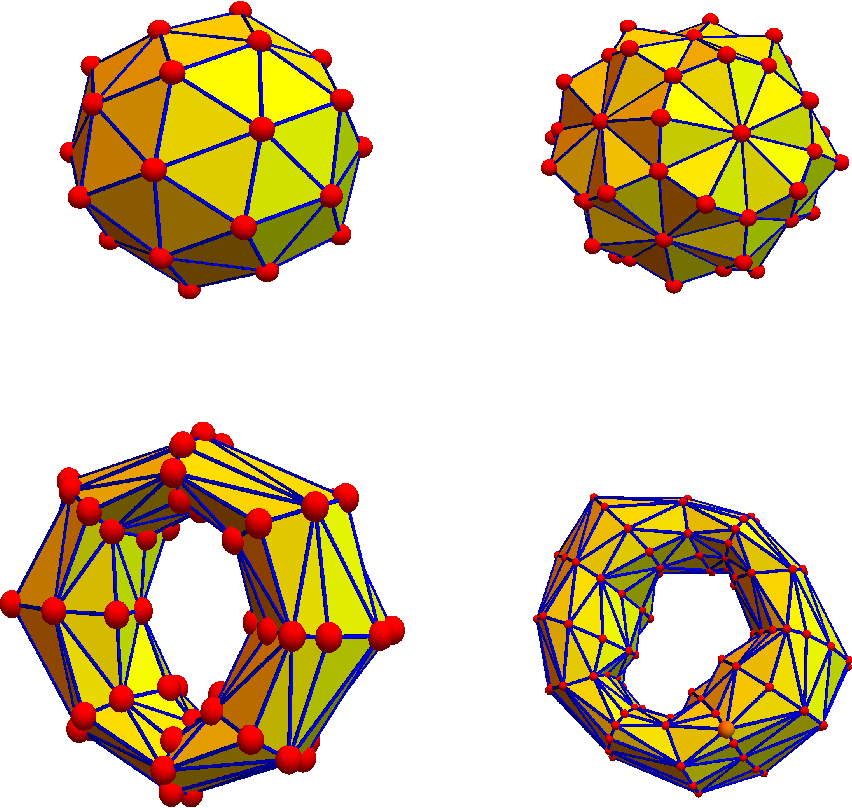•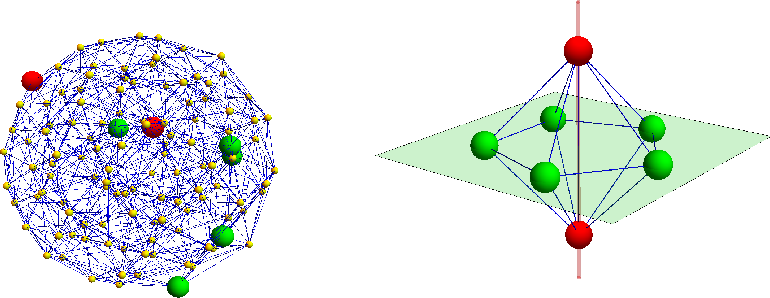•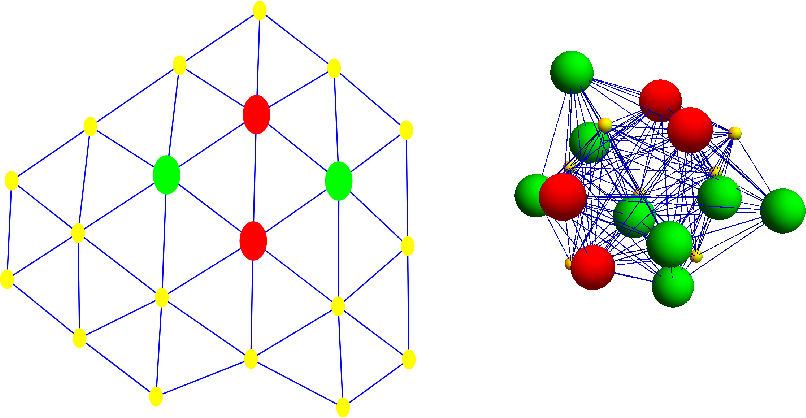•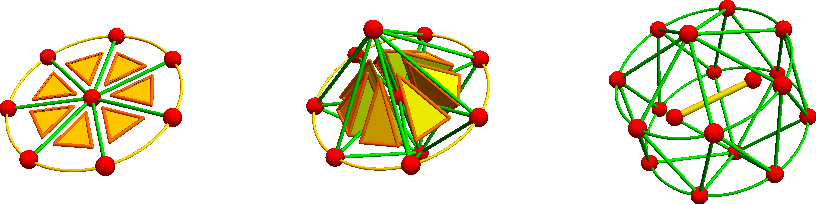•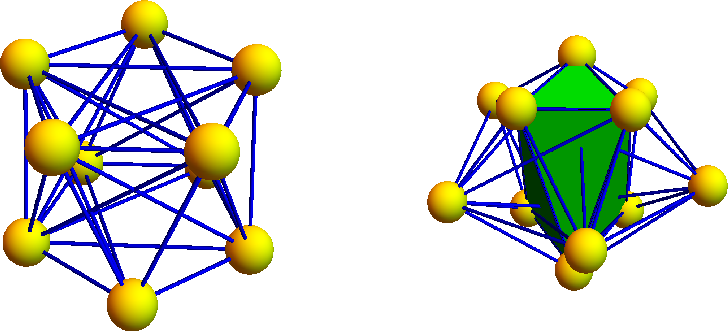2012
2012
• 2012
• Corpus ID: 124629033
(6) directed and undirected graph: A directed graph or digraph is consists of two finite sets, a set V (G) of vertices and a set… Expand
2003
2003
We introduce the idea of an n-simplex graph and games upon simplicial complexes. We then define moves on a labeled graph and pose… Expand
1998
1998